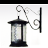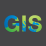# FEATURE CLASS FIELD CALCULATION BASED ON ANOTHER FIELD

939
4
10-17-2016 08:15 AMOccasional Contributor II

Hi,

I'm trying to calculate one field in the attribute table of a feature class based on another field with an if/else clause (please see script).

As a test, I used the values of "10" and "20" as results and it works. Now I need to replace that with two more complex expressions using other fields within the attribute table. That is:

Instead of "10" : 0.0006*B*(Q0.31) *(Area_km20.5) *(Z_MAX/1000)*MAT

and

Instead of "20" : 0.02B(Q)-0.31 *Area_km20.5 *(Z_MAX/1000)

"B"; "Q"; "Area_km2"; "Z_MAX"; "MAT" being those other fields in the attribute table.

I'm not quiet sure how to include those fields as well as the Power function within the codeblock part of the script.

Any help would be greatly appreciate it.

Many thanks

``````    # Add "BQART_QS" field to Nodes FC

BQART_QS_expression = "calc(!MAT!)"

codeblock = """def calc(val):
if val >= 2:
return 10
else:
return 20"""

arcpy.CalculateField_management(nodes, "BQART_QS", BQART_QS_expression, "PYTHON",codeblock)
‍‍‍‍‍‍‍‍‍‍‍‍‍``````
1 Solution

Accepted SolutionsbyEsri Esteemed Contributor

Hi AnToni,

You can use the math.pow method.  I believe you would have something like the following:

``````BQART_QS_expression = "calc(!B!, !Q!, !Area_km2!, !Z_MAX!, !MAT!)"

codeblock = """def calc(B, Q, Area_km2, Z_MAX, MAT):
if MAT >= 2:
return 0.0006*B*(math.pow(Q, -31)) *(math.pow(Area_km2, 0.5)) *(Z_MAX/1000)*MAT
else:
return 0.02*B*(math.pow(Q, -31)) *(math.pow(Area_km2, 0.5)) *(Z_MAX/1000)"""‍‍‍‍‍‍‍``````
4 RepliesbyEsri Esteemed Contributor

Hi AnToni,

You can use the math.pow method.  I believe you would have something like the following:

``````BQART_QS_expression = "calc(!B!, !Q!, !Area_km2!, !Z_MAX!, !MAT!)"

codeblock = """def calc(B, Q, Area_km2, Z_MAX, MAT):
if MAT >= 2:
return 0.0006*B*(math.pow(Q, -31)) *(math.pow(Area_km2, 0.5)) *(Z_MAX/1000)*MAT
else:
return 0.02*B*(math.pow(Q, -31)) *(math.pow(Area_km2, 0.5)) *(Z_MAX/1000)"""‍‍‍‍‍‍‍``````byMVP Esteemed Contributor

No need for a math module

``````>>> import math
>>> a = 2
>>> math.pow(a,2)
4.0
>>> a**2
4‍‍‍‍‍‍``````Occasional Contributor II

Thanks very much DanOccasional Contributor II

Thanks a lot Jake. That works perfect!